Next: Potential due to uniform Up: Newtonian gravity Previous: Potential due to uniform

# Potential outside uniform spheroid

Let us now calculate the gravitational potential generated outside a spheroid of uniform mass density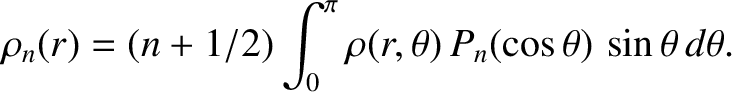and mean radius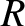. A spheroid is the solid body produced by rotating an ellipse about a major or a minor axis. Let the axis of rotation coincide with the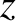-axis, and let the outer boundary of the spheroid satisfy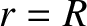(3.56)

where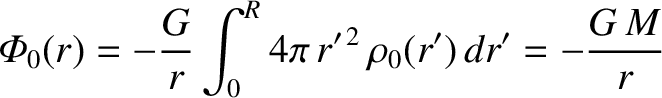is termed the ellipticity. In fact, the radius of the spheroid at the poles (i.e., along the rotation axis) is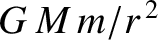, whereas the radius at the equator (i.e., in the bisecting plane perpendicular to the axis) is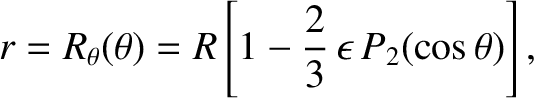. Hence,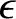(3.57)

Let us assume that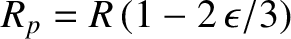, so that the spheroid is very close to being a sphere. If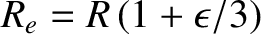then the spheroid is slightly squashed along its symmetry axis and is termed oblate. Likewise, if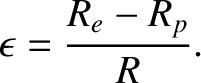then the spheroid is slightly elongated along its axis and is termed prolate. (See Figure 3.1.) Of course, if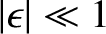then the spheroid reduces to a sphere.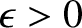Now, according to Equation (3.45) and (3.46), the gravitational potential generated outside an axially symmetric mass distribution can be written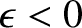(3.58)

where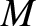is the total mass of the distribution, and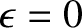(3.59)

Here, the integral is taken over the whole cross section of the distribution in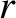-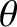space.

It follows that for a uniform spheroid, for which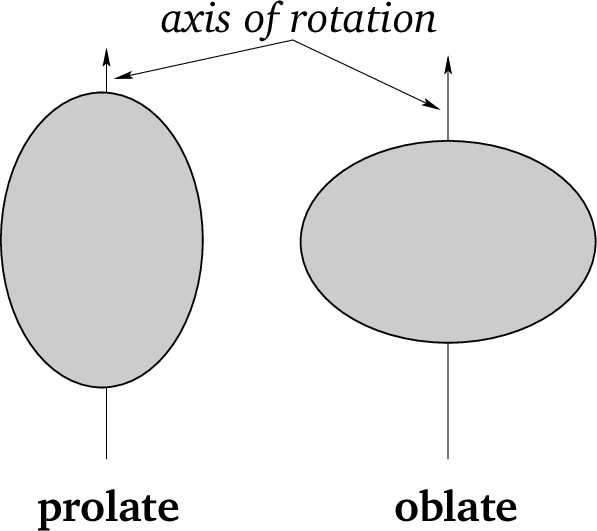,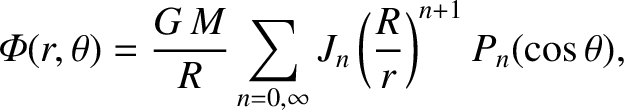(3.60)

Hence,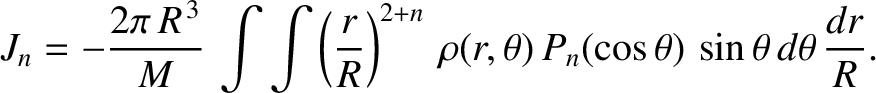(3.61)

giving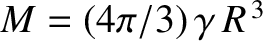(3.62)

to first order in. It is thus clear, from Equation (3.42), that, to first order in, the only nonzero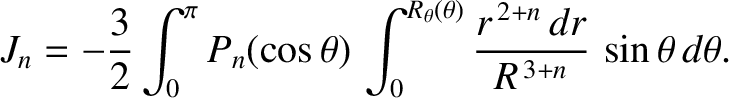are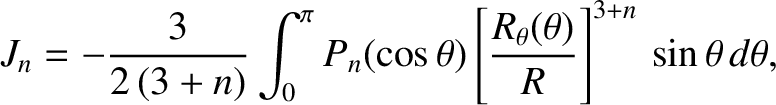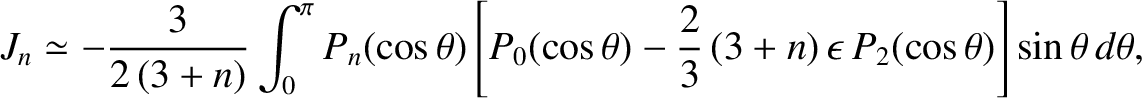(3.63) and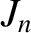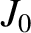(3.64)

Thus, the gravitational potential outside a uniform spheroid of total mass, mean radius, and ellipticityis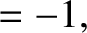(3.65)

By analogy with the preceding analysis, the gravitational potential outside a general (i.e., axisymmetric, but not necessarily uniform) spheroidal mass distribution of mass, mean radius, and ellipticity(where) can be written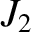(3.66)

where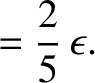. In particular, the gravitational potential on the surface of the spheroid is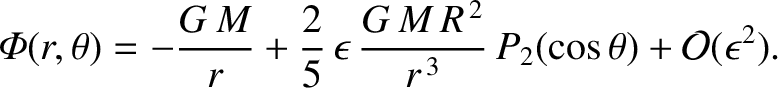(3.67)

which yields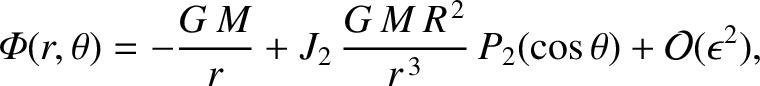(3.68)

where use has been made of Equation (3.56). For the case of a uniform-density spheroid, for which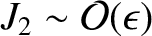[see Equation (3.64)], the preceding expression simplifies to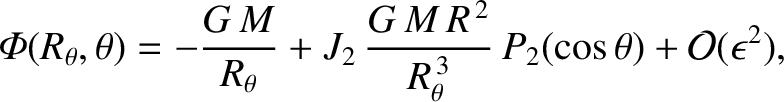(3.69)

Consider a self-gravitating spheroid of mass, mean radius, and ellipticity, such as a star or a planet. Assuming, for the sake of simplicity, that the spheroid is composed of uniform-density incompressible fluid, it follows that the gravitational potential on its surface is given by Equation (3.69). However, the condition for an equilibrium state is that the potential be constant over the surface. If this is not the case then there will be gravitational forces acting tangential to the surface. Such forces cannot be balanced by internal fluid pressure, which only acts normal to the surface. Hence, from Equation (3.69), it is clear that the condition for equilibrium is. In other words, the equilibrium configuration of a uniform-density, self-gravitating, fluid, mass distribution is a sphere. Deviations from this configuration can only be caused by forces in addition to self-gravity and internal fluid pressure; for instance, internal tensile forces, centrifugal forces due to rotation, and tidal forces due to orbiting masses. (See Chapter 6.) The same is true for a self-gravitating mass distribution of non-uniform density.

We can estimate how small a rocky asteroid, say, needs to be before its material strength is sufficient to allow it to retain a significantly non-spherical shape. The typical density of rocky asteroids in the solar system is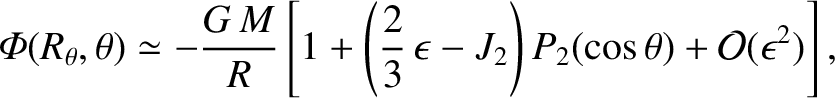. Moreover, the critical pressure above which the rock out of which such asteroids are composed ceases to act like a rigid material, and instead deforms and flows like a liquid, is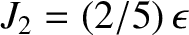(de Pater and Lissauer 2010). We must compare this critical pressure with the pressure at the center of the asteroid. Assuming, for the sake of simplicity, that the asteroid is roughly spherical, of radius, and of uniform density, the central pressure is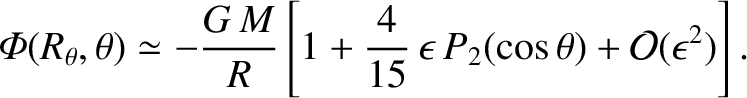(3.70)

where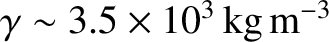is the gravitational acceleration at radius. [See Equation (3.53).] This result is a simple generalization of the well-known formula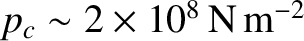for the pressure a depth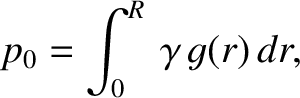below the surface of a fluid. It follows that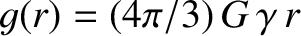(3.71)

If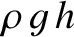then the internal pressure in the asteroid is not sufficiently high to cause its constituent rock to deform like a liquid. Such an asteroid can therefore retain a significantly non-spherical shape. On the other hand, if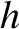then the internal pressure is large enough to render the asteroid fluidlike. Such an asteroid cannot withstand the tendency of self-gravity to make it adopt a spherical shape. The same applies to any rocky body in the solar system. The conditionis equivalent to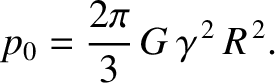, where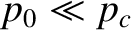(3.72)

It follows that only a rocky body whose radius is significantly less than about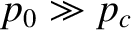--for instance, the two moons of Mars, Phobos (see Figure 3.2) and Deimos--can retain a highly non-spherical shape. On the other hand, a rocky body whose radius is significantly greater than about--for instance, the asteroid Ceres (see Figure 3.3) and the Earth's moon--is forced by gravity to be essentially spherical.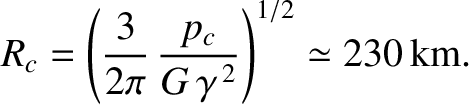According to Equations (3.58) and (3.63) , the gravitational potential outside a general axisymmetric body of massand mean radiuscan be written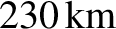(3.73)

where theare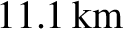(or smaller) dimensionless parameters that depend on the exact shape of the body. However, a long way from the body (i.e.,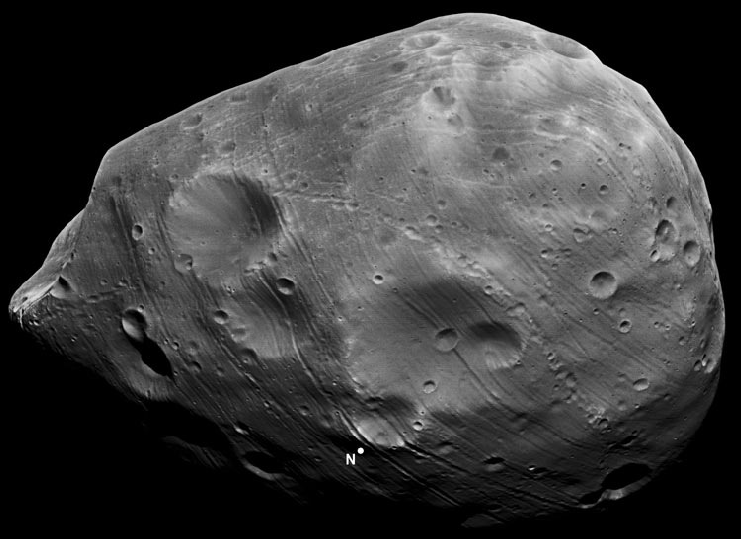), the right-hand side of the preceding expression is clearly dominated by the first term inside the round brackets, so that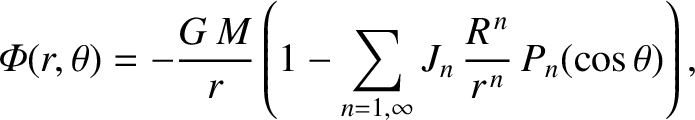(3.74)

However, this is just the gravitational potential of a point particle, located at the center of the body, whose mass is equal to that of the body. This suggests that the gravitational interaction between two irregularly shaped bodies in the solar system can be approximated as the interaction of two point masses, provided that the distance between the bodies is much larger than the sum of their radii.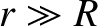Next: Potential due to uniform Up: Newtonian gravity Previous: Potential due to uniform
Richard Fitzpatrick 2016-03-31